## Goldbach conjecture ★★★★

Author(s): Goldbach

Conjecture   Every even integer greater than 2 is the sum of two primes.

## The Erdos-Turan conjecture on additive bases ★★★★

Author(s): Erdos; Turan

Let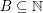. The representation function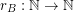foris given by the rule. We callan additive basis if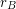is never.

Conjecture   Ifis an additive basis, thenis unbounded.

## The Alon-Tarsi basis conjecture ★★

Author(s): Alon; Linial; Meshulam

Conjecture   Ifare invertible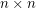matrices with entries in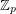for a prime, then there is asubmatrixof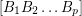so thatis an AT-base.

Conjecture   For every prime, there is a constant(possibly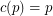) so that the union (as multisets) of anybases of the vector spacecontains an additive basis.